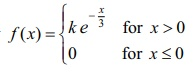Home | | Maths 12th Std | Exercise 11.3: Continuous Distributions

# Exercise 11.3: Continuous Distributions

Maths Book back answers and solution for Exercise questions - Mathematics : Probability Distributions: Continuous Distributions - Exercise Problem Questions with Answer, Solution

EXERCISE 11.3

1. The probability density function of X is given byFind the value of k .2. The probability density function of X isFind (i) P (0.2 ≤ X < 0.6) (ii) P (1.2 ≤ X < 1.8) (iii) P (0.5 ≤ X < 1.5)3. Suppose the amount of milk sold daily at a milk booth is distributed with a minimum of 200 litres and a maximum of 600 litres with probability density functionFind (i) the value of (ii) the distribution function (iii) the probability that daily sales will fall between 300 litres and 500 litres?4. The probability density function of X is given byFind (i) the value of (ii) the distribution function (iii) P(< 3) (iv) (5  X ) (v) P(≤ 4).5. If X is the random variable with probability density function f ( x) given by,then find (i) the distribution function F ( x) (ii) P ( 0.5 ≤ X ≤ 0.5)6. If X is the random variable with distribution function F ( x) given by,then find (i) the probability density function f ( x) (ii) P ( 0 .3  X  0 .6)